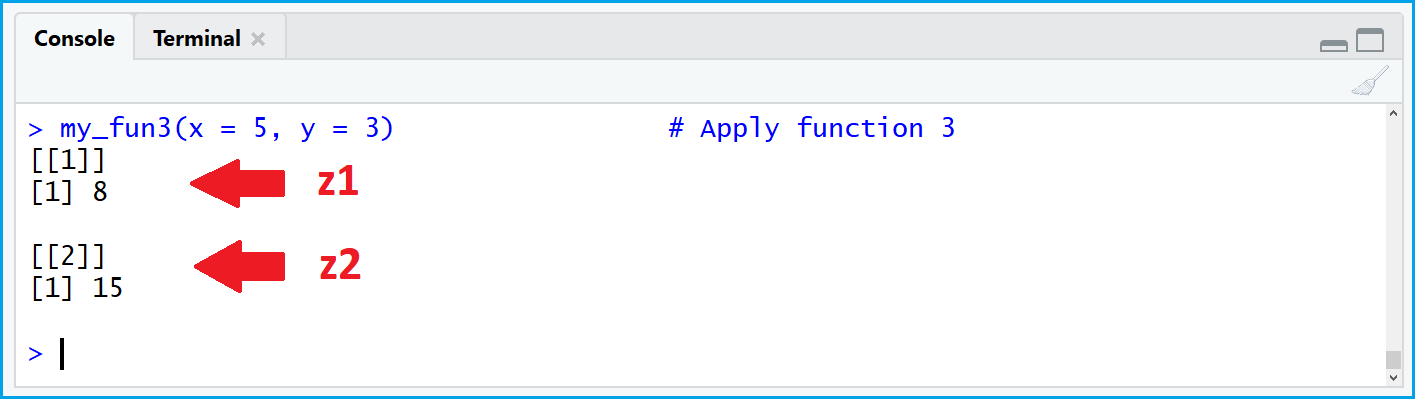# Return Value from R Function (3 Examples)

This article shows how to apply the return command to produce outputs with user-defined R functions. The article contains three reproducible examples:

Let’s dive in!

## Example 1: R Function with return

This example shows a simple user-defined R function, which computes the sum of the two input values x and y.

The last row of code shows how to use the return command in R. We simply need to insert the desired output of our function between the parentheses of the return command:

```my_fun1 <- function(x, y) {           # R function with return
z <- x + y
return(z)
}```

After running the previous R syntax, we can apply our user-defined function as follows:

```my_fun1(x = 5, y = 3)                 # Apply function 1
# 8```

We used the input values 5 and 3 and our function returned the value 8 (i.e. 5 + 3 = 8).

Looks good!

However, is the return command really needed? That’s what you will learn in the next example.

## Example 2: R Function without return

Let’s delete the return command from our function of Example 1…

```my_fun2 <- function(x, y) {           # R function without return
z <- x + y
z
}```

…and the let’s apply it again:

```my_fun2(x = 5, y = 3)                 # Apply function 2
# 8```

Same output!

Question: Why do we need the return command?

Answer: R returns the last output of a function automatically. We therefore do not need to use the return explicitly.

However, using the return command is often considered as good practice, since it makes the R code easier to read and understand.

This is especially the case in more complex functions, e.g. when we are returning multiple values as a list…

## Example 3: Return Multiple Values as List

For illustration, I will show you a slightly more complex example for the usage of return in R. Consider the following function:

```my_fun3 <- function(x, y) {           # Return multiple values
z1 <- x + y
z2 <- x * y
return(list(z1, z2))
}```

If we apply the function, we get the following list output:

`my_fun3(x = 5, y = 3)                 # Apply function 3`Figure 1: Multiple Function Outputs Returned as List.

The more complex our function gets, the more helpful is the return command. We could simply go back to our function and search for return( to get a quick overview of our output.

Therefore, I recommend to use return in every user-defined function. It’s not much programming work, but makes our lives much easier!

## Tutorial Video & Further Resources

If you still have questions concerning the method I used in this tutorial, feel free to watch the video on my YouTube channel, where I describe the syntax more detailed:

Please accept YouTube cookies to play this video. By accepting you will be accessing content from YouTube, a service provided by an external third party.If you accept this notice, your choice will be saved and the page will refresh.

Please accept YouTube cookies to play this video. By accepting you will be accessing content from YouTube, a service provided by an external third party.If you accept this notice, your choice will be saved and the page will refresh.

Furthermore, you might want to have a look at the other R tutorials on my website:

At this point, you should have learned why and when to use the return command in R.

As a final note: In this tutorial we have returned a single value from our user-defined function. However, it would also be possible to use a similar R syntax to return other types of data objects such as a data frame, a tibble, a ggplot2 plot object, and so on…

Just leave me a comment below in case you have any further questions.

Subscribe to the Statistics Globe Newsletter

•Noah
April 7, 2021 9:26 am

•April 7, 2021 9:26 am

Thanks a lot Noah, glad to hear that! 🙂

•Per
December 2, 2021 9:46 am

Hi!! Stumbled across this and found it very helpful. One thing i missed though was a total sum of principal over term.

•December 2, 2021 10:34 am

Hi Per,

Thanks a lot for the kind comment, glad you found it helpful! Could you explain what you mean with “total sum of principal over term”?

Regards,
Joachim

•Hossain Shaikhani
May 26, 2022 6:48 pm

Your explanations and examples are illustrative and easy to understand. Thank you.

•June 6, 2022 4:24 pm

Thank you very much Hossain, this is great to hear!

Regards,
Joachim

•George
March 15, 2023 3:25 pm

However, I would like to ask if there’s a way to create a function that returns individual values for a data frame or list.
Thank you

•March 16, 2023 10:26 am